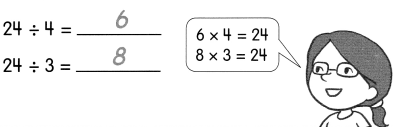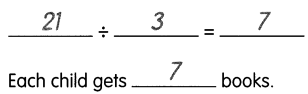# Math in Focus Grade 2 Chapter 15 Practice 5 Answer Key Divide Using Related Multiplication Facts

This handy Math in Focus Grade 2 Workbook Answer Key Chapter 15 Practice 5 Divide Using Related Multiplication Facts detailed solutions for the textbook questions.

## Math in Focus Grade 2 Chapter 15 Practice 5 Answer Key Divide Using Related Multiplication Facts

Complete the multiplication sentences. Then complete the division sentences.

ExampleQuestion 1.
4 × ____________ = 8
____________ × 4 = 8
8 ÷ 2 = ____________
8 ÷ 4 = ____________

4 × 2 = 8
2 × 4 = 8
8 ÷ 2 = 4
8 ÷ 4 = 2

Question 2.
4 × ____________ = 12
____________ × 4 = 12
12 ÷ 3 = ____________
12 ÷ 4 = ____________

4 × 3 = 12
3 × 4 = 12
12 ÷ 3 = 4
12 ÷ 4 = 3

Question 3.
5 × ____________ = 15
____________ × 5 = 15
15 ÷ 3 = ____________
15 ÷ 5 = ____________

5 × 3 = 15
3 × 5 = 15
15 ÷ 3 = 5
15 ÷ 5 = 3

Question 4.
5 × ____________ = 20
____________ × 5 = 20
20 ÷ 4 = ____________
20 ÷ 5 = ____________

5 × 4 = 20
4 × 5 = 20
20 ÷ 4 = 5
20 ÷ 5 = 4

Use related multiplication facts to solve.

Example
The teacher divides 21 books equally among 3 children. How many books does each child get?Question 5.
Mr. Holtz gives $24 to 4- workers. The workers share the money equally among themselves. How much money does each worker get?$____________ ÷ ____________ = $____________ Each worker gets$____________.

$24 ÷ 4 =$6

Each worker gets \$6.

Question 6.
Rita has 27 stuffed toys. She puts them on 3 shelves. She puts the same number on each shelf. How many stuffed toys are on each shelf?
____________ ÷ ____________ = ____________
There are ____________ stuffed toys on each shelf.

27 ÷ 3 = 9

There are 9 stuffed toys on each shelf.

Use related multiplication facts to solve.

Question 7.
Phil puts 36 pencils equally into 4 boxes. How many pencils are in each box?
____________ ÷ ____________ = ____________
There are ____________ pencils in each box.

36 ÷ 4 = 9

There are 9 pencils in each box.

Question 8.
Angie uses 9 craft sticks to make 3 triangles of the same size. How many craft sticks are needed to make one triangle?
____________ ÷ ____________ = ____________
____________ craft sticks are needed to make one triangle.

9 ÷ 3 = 3

3 craft sticks are needed to make one triangle.

Question 9.
Sammy fixes 8 tires on his cars. He fixes 4 tires on each car. How many cars are there?
____________ ÷ ____________ = ____________
There are ____________ cars.

8 ÷ 4 = 2

There are 2 cars.

Question 10.
Mr. Yuma has 18 pieces of bread. He puts 3 pieces in each basket. How many baskets does he use?
____________ ÷ ____________ = ____________

18 ÷ 3 = 6

Question 11.
A photo album contains 20 photos. Each filled page of the album has 4 photos. How many pages are filled?
____________ ÷ ____________ = ____________
____________ pages are filled.

20 ÷ 4 = 5

5 pages are filled.

Question 12.
Keisha has 9 fish. She puts 3 fish in each fish tank. How many fish tanks does she need?
____________ ÷ ____________ = ____________
She needs ____________ fish tanks.